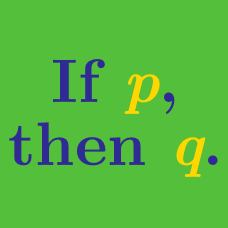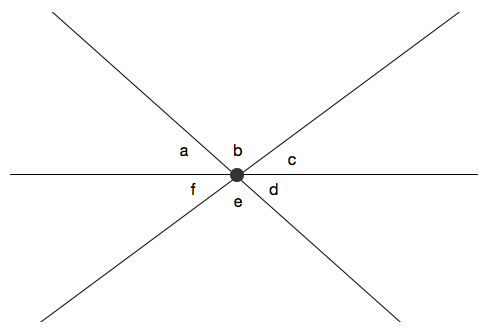Everyday Math

# Irrelevant Information

Sarah has $17$ cousins and $3$ sisters. If she and her sisters want to take $4$ other boys to the dance, in how many ways can they be paired up in boy-girl pairs?

(A) $\ \ 3$
(B) $\ \ 4$
(C) $\ \ 6$
(D) $\ \ 17$
(E) $\ \ 24$The above diagram shows three straight lines intersecting at a point. If $a = 27 ^ \circ, b = 101 ^ \circ,$ and $c = 52^\circ,$ what is the value of $e?$

(A) $\ \ 27$
(B) $\ \ 52$
(C) $\ \ 79$
(D) $\ \ 101$
(E) $\ \ 128$

Jane went grocery shopping with her mom and bought 7 cucumbers for thirty cents each, a bunch of bananas for two dollars and eighty cents, and 5 apples for one dollar and twenty cents. How much would it cost (in cents) to buy one apple and one cucumber at this grocery store?

(A) $\ \ 31$
(B) $\ \ 54$
(C) $\ \ 127$
(D) $\ \ 150$
(E) $\ \ 330$

Kevin bought $a$ apples at $\ 0.80$ each. He also bought $b$ bunches of bananas; the bananas cost a total of $\5.50.$ How much did he spend for the apples and the bananas?

(A) $\ \ \ 0.80 + \ 5.50$
(B) $\ \ \0.80 \times a + \ 5.50$
(C) $\ \ \ 0.80 + \5.50 \times b$
(D) $\ \ \0.80 \times a + \5.50 \times b$
(E) $\ \ \0.80 \times a \times \5.50 \times b$

S&P 500 index closed at 2000 on Friday last week, and it went up by 20 points on Monday. The index lost 30 points the next day and closed at 2040 on Wednesday. Then it went up by 40 points on Thursday and closed the week gaining another 40 points on Friday, hitting a record high. What was the change in the index on Wednesday?

(A) $\ \ -10$
(B) $\ \ 40$
(C) $\ \ 50$
(D) $\ \ 80$
(E) $\ \ 120$

×